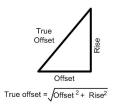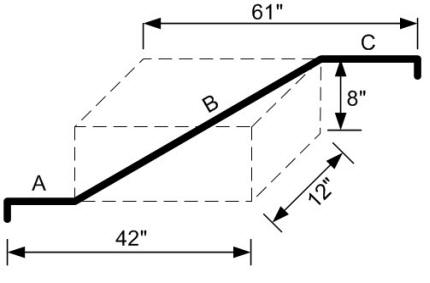# Rolling Offset Formula

A rolling offset in a plumbing system is when a pipe changes in both the horizontal and vertical planes. To visualize the travel of the pipe, imagine a three dimensional box with the pipe entering at one corner and exiting at the farthest diagonal corner.##### Step 1 - Calculating the True OffsetThe first number you need to find when calculating a rolling offset is the "true offset" which is found using Pythagoras' theorem. This simply means that the offset squared plus the rise squared will equal the true offset squared. You then need to take the square root of the result to get the true offset.

##### Step 2 - Finding the Setback and Diagonal

Once you know the true offset you can use a table to determine the setback and diagonal center to center measurements. See the table below for the most common fitting constants. If your studying for a plumbing exam you will need to remember the 45˚ constants.

 Fitting angle60˚45˚22.5˚ Diagonal = true offset X1.1551.4142.613 Setback = true offset X0.5771.0002.414

### Practice Question

Solve for pipes A, B, and C in the image below using 45 degree fittings and again using 22.5 degree fittings. The measurements are shown in imperial but all the constants are the same for metric. Scroll down for answers.Solve for...22.5 Degree fitting45 Degree fitting True offset14.42"14.42" Setback34.81"14.42" Pipe A42" - setback =7.19"27.58" Pipe B37.68"20.39" Pipe C61" - setback =26.19"46.58"

# These Articles May Also Be Helpful:

 Formula: Pressure InDepths Of LiquidHow To Size Water LinesJumper OffsetRoof Drain CalculationsPlumbing Math Formulas